# Stereo

How to deal with stereo in NUKE.

## Multi View Knob Values

Adding values per view in a stereo project is as simple as adding different values to a translate’s x and y knob. Here is a quick primer.

This adds a Transform node and splits out the right view:

```n = nuke.createNode('Transform')
k = n['translate']
k.splitView('right')
```

When splitting out a view, all “un-split” views are controlled together like a “main” view, so if no explicit view is specified, all “un-split” knobs are changed.

The following assigns the value of 1 to the knob’s x and y field for the main view, but not for the split out views:

```k.setValue(1)
```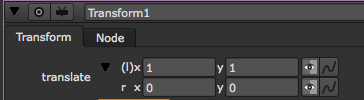This assigns the value of 2 to only the right view:

```k.setValue(2, view='right')
```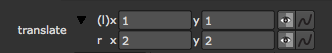This sets the translate values for the main view to x=1 and y=2:

```k.setValue(1, 0)
k.setValue(2, 1)
```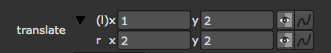While this sets the translate values in the right view to x=3 and y=4:

```k.setValue(3, 0, view='right')
k.setValue(4, 1, view='right')
```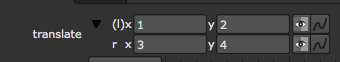You can create animation in different views in a similar way. This sets the main views to be animated:

```k.setAnimated()
```

This sets the right view to be animated:

```k.setAnimated(view='right')
```

Setting keyframes for main and right view at frame 1 and 100:

```k.setValueAt(50, 1)
k.setValueAt(70, 100)

k.setValueAt(80, 1, view='right')
k.setValueAt(110, 100, view='right')
```

And reading animation values for both views:

```mainX = k.valueAt(50, 0)
mainY = k.valueAt(50, 1)
rightX = k.valueAt(50, 0, view='right')
rightY = k.valueAt(50, 1, view='right')

print 'left (main) view values at frame 50 are X=%s, Y=%s' % (mainX, mainY)
print 'right view values at frame 50 are X=%s, Y=%s' % (rightX, rightY)
```
`# Result:`
`left (main) view values at frame 50 are X=59.898989898, Y=59.898989898`
`right view values at frame 50 are X=94.8484848507, Y=94.8484848507`

## Examples

### Creating/Converting a Stereo Camera

Here is an example function to create or convert a camera for stereo work. Start with the usual function definition using two arguments to define the Camera node and the interocular distance. Set the default value for node to None (in which case we create a new camera) and the interoc to whatever value works for you:

```def stereoCam(node=None, interoc=.6):
try:
node = node or nuke.selectedNode()
except ValueError:
# IF NO NODE IS GIVEN AND NOTHING IS SELECTED, CREATE A NEW NODE
node = nuke.createNode( 'Camera2' )
```

Next we get the current script’s views. We assume the first view in the list is the left eye, the second the right eye:

views = nuke.views() leftView = views rightView = views

Calculate the offset from the camera’s current position to the left and right based on the given interoc value:

```rightOffset = float(interoc)/2
leftOffset = -rightOffset
```

The camera can be driven by the matrix knob or by the transform knobs. Let’s start with the first case - grab the matrix knob and copy the current matrix so we can create the second eye with it:

```if node['useMatrix'].value():
knob = node['matrix']
leftEyeMatrix = node['transform'].value() # GETS MATRIX BUT IN REVERSE ORDER
rightEyeMatrix = nuke.math.Matrix4(leftEyeMatrix) # COPY MATRIX
```

Now move the original matrix to the left to represent the left eye based on the values calculated above, then do the same for the right eye:

```leftEyeMatrix.translate(leftOffset, 0, 0)
rightEyeMatrix.translate(rightOffset, 0, 0)
```

Because node[‘transform’].value() above returned the matrix in reverse order, we need to reverse it again before we assign the new values:

```leftEyeMatrix.transpose()
rightEyeMatrix.transpose()
```

If there are only two views in the script, we use the left view as the main view and only split off the right view. If there are more than two views we split both:

```if len(views) > 2:
knob.splitView(leftView)
knob.splitView(rightView)
```

Now we can assign the new values by cycling through the values of the 4x4 matrix and assigning them one by one:

```for i in range(16):
knob.setValueAt(leftEyeMatrix[i], nuke.frame(), i, leftView)
knob.setValueAt(rightEyeMatrix[i], nuke.frame(), i, rightView)
```

This takes care of the case where the camera is driven by the matrix knob. In the other case, things are a little easier but we are doing the same stuff. Grab the transform knob’s x value and offset it by the values calculated from the interocular argument:

```else:
knob = node['translate']
# GET THE NEW VALUES FOR LEFT AND RIGHT EYE
leftEye = knob.value(0) + leftOffset
rightEye = knob.value(0) + rightOffset
```

Split the knobs as required:

```if len( views ) > 2:
knob.splitView( leftView )
knob.splitView( rightView )
```

Assign the new values to the new views:

```knob.setValue( leftEye, 0, view=leftView )
knob.setValue( rightEye, 0, view=rightView )
```

The final code:

```import nuke

def stereoCam( node=None, interoc=.6 ):
'''
Create a simple stereo camera or convert an existing one.
args:
node - camera node to convert to stereo. if None a camera will be created
interoc  -  distance between right and left view
'''
try:
node = node or nuke.selectedNode()
except ValueError:
# IF NO NODE IS GIVEN AND NOTHING IS SELECTED, CREATE A NEW NODE
node = nuke.createNode( 'Camera2' )

# GET SCRIPT SETTIONGS' VIEWS
views = nuke.views()
leftView = views
rightView = views

# THE OFFSET AS REQUESTED
rightOffset = float(interoc)/2
leftOffset = -rightOffset

# THE KNOB TO SPLIT
if node['useMatrix'].value():
knob = node['matrix']
leftEyeMatrix = node['transform'].value() # GETS MATRIX BUT IN REVERSE ORDER
rightEyeMatrix = nuke.math.Matrix4( leftEyeMatrix ) # COPY MATRIX

# GET THE NEW VALUES FOR LEFT AND RIGHT EYE
leftEyeMatrix.translate( leftOffset, 0, 0 )
rightEyeMatrix.translate( rightOffset, 0, 0 )

# REVERSE FOR ASSIGNMENT
leftEyeMatrix.transpose()
rightEyeMatrix.transpose()

# IF THERE ARE MORE THAN 2 VIEWS MAKE SURE TO SPLIT OFF LEFT VIEW AS WELL
if len( views ) > 2:
knob.splitView( leftView )
knob.splitView( rightView )

# ASSIGN VALUES
for i in range(16):
knob.setValueAt( leftEyeMatrix[i], nuke.frame(), i, leftView )
knob.setValueAt( rightEyeMatrix[i], nuke.frame(), i, rightView )
else:
knob = node['translate']
# GET THE NEW VALUES FOR LEFT AND RIGHT EYE
leftEye = knob.value(0) + leftOffset
rightEye = knob.value(0) + rightOffset

# IF THERE ARE MORE THAN 2 VIEWS MAKE SURE TO SPLIT OFF LEFT VIEW AS WELL
if len( views ) > 2:
knob.splitView( leftView )
knob.splitView( rightView )

# ASSIGN NEW VALUE
knob.setValue( leftEye, 0, view=leftView )
knob.setValue( rightEye, 0, view=rightView )

```

### Setting Up Stereo

Here is a way to automatically force new NUKE projects to be stereo (or generally multi view) setups. First, we write a function that turns a NUKE project into a multi view project with all the desired parameters, then we hook up that function to a callback making sure it is run every time a new root node is created.

The knob we need to modify to create new views is nuke.root().knob(‘views’). This knob does not have any dedicated methods to set views, so let’s examine it’s script syntax for a default stereo project:

```viewKnob = nuke.root().knob('views')
print viewKnob.toScript()

# Result:
left #ff0000
right #00ff00
```

The toScript method converts the knob to it’s script syntax, the way it’s written in the .nk file, and is a good way to analyse complex knobs. In this case, it tells us the root’s view names and associated colors in hex code. The views are delimited by line breaks. With this info we can now construct our own list of names and color values, then use the fromScript method to initialize the knob with our values.

Let’s test this first:

```viewKnob = nuke.root().knob('views')
viewKnob.fromScript( 'testView #ff0000')
```
This sets the root’s view knob to have a single view called testView and assigns a primary red to it.
In reality, it is much more convenient to be able to assign rgb values rather than hex values, so let’s write a little converter to avoid having to worry about hex values in the future - using Python’s string formatting this is pretty simple.

This converts an integer value to a hex value:

```intValue = 255
hexValue = '%x' % intValue
print hexValue

# Result:
ff
```

So let’s do this for r, g and b:

```rgb = (255, 0, 0)
hexCol = '#%02x%02x%02x' % rgb
print hexCol

# Result:
#ff0000
```

Since NUKE mostly works in floating point values, let’s modify this so we can input normalized values (0-1) rather than 8 bit integers (0-255):

```col = (.5,.3,.2)
rgb = tuple([ int(v*255) for v in col ])
hexCol = '#%02x%02x%02x' % rgb
print hexCol

# Result:
#7f4c33
```

The above converts each normalized floating point value in col to an 8 bit integer using list comprehension. The output is a list which is converted to a tuple before being stored in rgb - this is essential for the subsequent string formatting requirements.

Let’s throw it all together to build our own script syntax for a custom view called testView and assign a dark red color:

```name = 'testView'
col = (.5, 0, 0)
rgb = tuple([ int(v*255) for v in col ])
hexCol = '#%02x%02x%02x' % rgb
view = '%s %s' % (name, hexCol)
# NOW INITIALIZE THE VIEW KNOB WITH THE NEW VIEW
nuke.root().knob('views').fromScript(view)
```

If we want more than one view, we just throw a line break in between the views:

```newViews = []

name = 'testView'
col = (.5, 0, 0)
rgb = tuple([ int(v*255) for v in col ])
hexCol = '#%02x%02x%02x' % rgb
view = '%s %s' % (name, hexCol)
newViews.append(view)

name2 = 'testView2'
col2 = (0, .5, 0)
rgb2 = tuple([ int(v*255) for v in col2 ])
hexCol2 = '#%02x%02x%02x' % rgb2
view2 = '%s %s' % (name2, hexCol2)
newViews.append(view2)

nuke.root().knob('views').fromScript('\n'.join(newViews))
```

Now let’s tidy this up, add the nuke import, and make a function that let’s the user define a list of views where each view is a tuple with the view’s name followed by it’s color:

```import nuke

def setUpMultiView( views=[ ('left',(0,1,0)), ('right',(1,0,0) ) ] ):
'''
set up the nuke project with an arbitrary amount of colour coded views
args:
views  -  nested list with view names and rgb tuples for each view. rgb values are assumed to be normalise, eg red = (1,0,0)
'''
newViews = []
for v in views:   # CYCLE THROUGH EACH REQUESTED VIEW
name = v   # GRAB THE CURRENT VIEWS NAME
col = v    # GRAB THE CURRENT VIEWS COLOUR
rgb = tuple( [ int(v*255) for v in col ] ) #CONVERT FLOAT TO 8BIT INT AND RETURN A TUPLE
hexCol = '#%02x%02x%02x' % rgb             #CONVERT INTEGER NUMBERS TO HEX CODE
curView = '%s %s' % ( name, hexCol )       #COMBINE NAME AND HEX COLOUR TO SCRIPT SYNTAX
newViews.append( curView )      # COLLECT ALL REQUESTED VIEWS

# COMBINE ALL VIEWS WITH LINE BREAK AND INITIALISE THE VIEWS KNOB WITH THE RESULTING SCRIPT SYNTAX
nuke.root().knob('views').fromScript( '\n'.join( newViews ) )
```
Run the function to test it. Note that the above function defines the left and right views with green and red colors as the default, so you could run the function without any arguments as well if you’re happy with those settings::

setUpMultiView()

If you want the left view to be red and the right one to be green, call the function with different values:

```setUpMultiView([ ('left', (1,0,0)), ('right', (0,1,0)) ])
```

The latter creates two views called left and right and assigns a primary red and green respectively.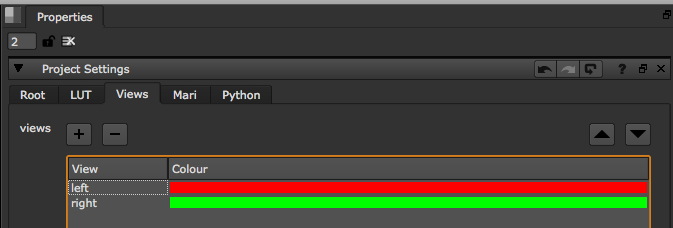All the hard work is done.

If you want NUKE to run this script on start-up, so that new sessions are automatically in stereo mode, hook up the function to the onUserCreate callback.